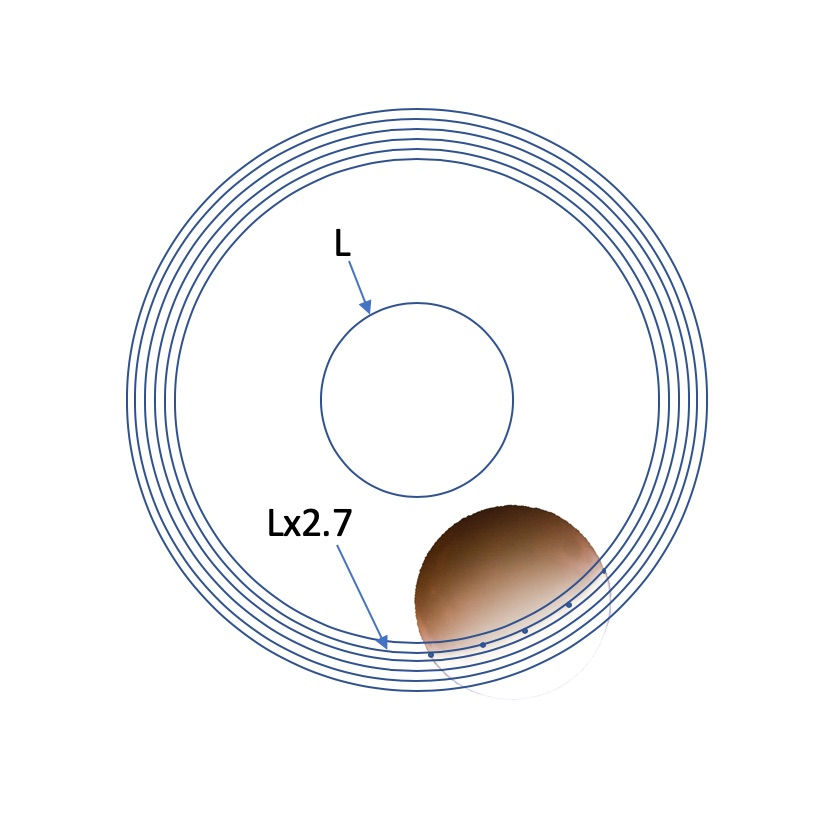Buscar
•Admin

# Calculating the size of the Moon with a photo

Actualizado: 2 abr 2020

Already Hipparchus of Nicea around 150 B.C. relied on the shadow of the Earth on the Moon during lunar eclipses to calculate the relative size of the Moon relative to the Earth, and deduced that it was 3.7 times smaller. The data is very similar to the one currently being handled, although Hipparco made several mistakes, but some compensated others and obtained a value very similar to the correct one.

One of Hipparchus errors was to estimate that the Sun was far enough away to be able to consider that the umbra during the eclipse coincided with the diameter of the Earth. This is not correct, as the size of the area of penumbra that occurs must also be considered since the Sun's rays do not reach the Earth parallel as if it were a source in infinity. The correct thing is to consider that the diameter of the Earth coincides with the equidist line between the umbra and the end of the penumbra.The tip line corresponds to the size of the Earth

From a photo of the lunar eclipse of July 16, 2019 we will calculate the diameter of the Moon (in proportion to that of the Earth) based on a purely geometric exercise. It's not a very accurate method, but it works.Picture of the Moon on July 16, 2019 from Majadahonda, Madrid

We draw a circle the same size as the moon (L). Next, we draw concentric circles with sizes proportional to L, for example, Lx2, Lx2.1, ... Lx3.

In the drawing we have only represented for clarity from Lx2.6 to Lx3. We place the Moon so that the line that marks the shadow of the Earth (umbra) during the eclipse coincides with one of the concentric circles, finding that the one that best coincides is L*2.7 (or a value between 2.7 and 2.8). That is, the umbra produced by the Earth during the eclipse is approximately 2.7 times the diameter of the Moon.We draw a circle the same size as the moon (L). Next, we draw concentric circles with sizes proportional to L, for example, Lx2, Lx2.1, ... Lx3.

In the drawing we have only represented for clarity from Lx2.6 to Lx3. We place the Moon so that the line that marks the shadow of the Earth (umbra) during the eclipse coincides with one of the concentric circles, finding that the one that best coincides is L*2.7 (or a value between 2.7 and 2.8). That is, the umbra produced by the Earth during the eclipse is approximately 2.7 times the diameter of the Moon.

To calculate the size of the Moon, we have to do these calculations:

Earth Diameter (T)= Umbra + Penumbra Band

And therefore:

T = 2.7xL + Penumbra Band

We used an astronomical coincidence to calculate the penumbra band. As we know, the apparent diameter of the Sun is practically the same as that of the Moon, and thanks to this we can enjoy impressive solar eclipses. But the band of penumbra corresponds to the diameter of the Sun seen from the Moon, which in turn coindices with the diameter of the Moon from the Earth.We can therefore write the above formula as:

T = 2.7xL + L = 3.7L

That is to say, T/L=3.7, and calculating it with the currently admitted values:

T/L=12742 km /3474 km=3.66

The real value differs less than 2% from the value we have calculated of 3.7 from a photo

It's amazing how much information a simple eclipse photo hides!

#EN

90 visualizaciones0 comentarios